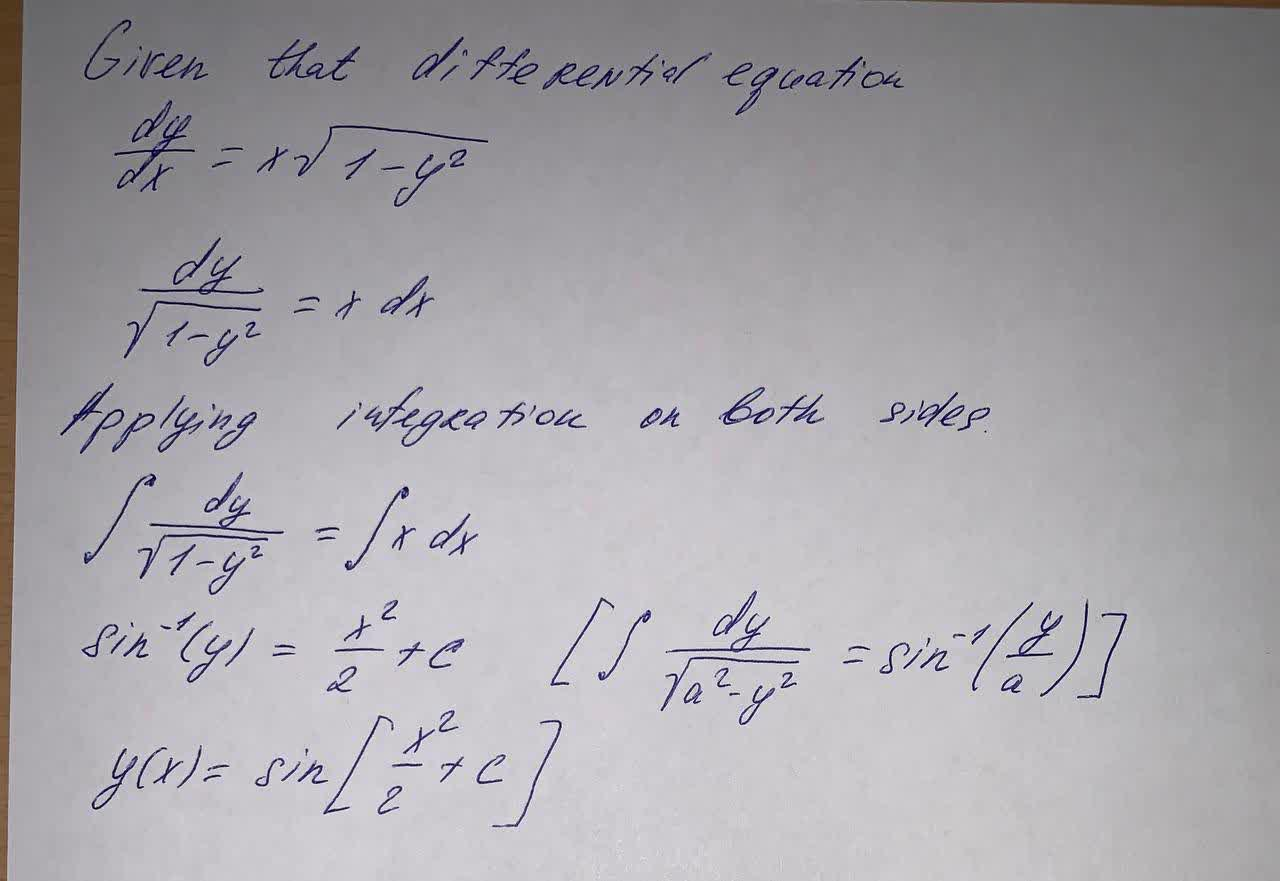Question# Solve the given differential equation by separation of variables: \frac{dy}{dx}=x\sqrt{1-y^2}

Differential equations
ANSWEREDSolve the given differential equation by separation of variables: $$\frac{dy}{dx}=x\sqrt{1-y^2}$$